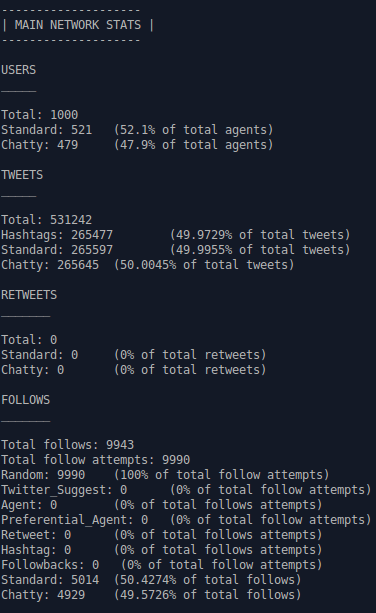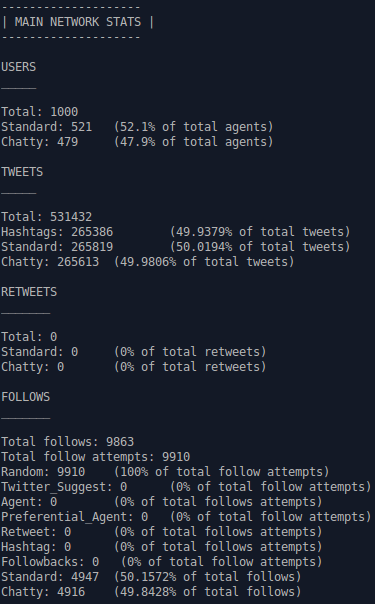hashkat.org

# Unfollowing

In graph theory unfollowing is the act of edge deletion. In #k@ it is when an agent stops following another agent.

In #k@ the 'reason' that an agent unfollows another agent is due to chattiness, or signal frequency exceeding a user given threshold. The threshold may be defined two ways, absolutely, based on the agent's tweet rate, and relatively, based on the receiver's receipt rate.

• unfollow tweet rate (absolute, related to sender)

In this tutorial we will discuss how unfollowing may be implemented in your network simulation. This tutorial should take approximately 20 minutes to complete.

The unfollow_tweet_rate is a user defined maximum tweet rate per minute. If an agent has a tweet rate greater than this number, the agent will be unfollowed by one of its followers, chosen randomly. That is, the 'chatty' agent, Agent A, who surpassed the tweet threshold rate, will have their in-degree lowered by one. Agent A's chattiness is defined based a number set for all agents in the simulation. The follower who is dropped is chosen at random from among Agent A's followers.

#### Stage 1 Unfollow

If stage1_unfollow is set to true into a simulation, the 'chatty' agent may also be defined NOT based on the agent's tweet rate, but based how much the agent tweets compared to the average tweet rate of all the other agents the following agent following. If the agent's tweet rate is greater than twice the average tweet rate of the other agents the follower follows, then the follower will unfollow the chatty agent.

As an example, Agent E follows Agents B, C, and D. Agents B, C and D tweet at an average rate of 5 tweets per minute per agent. Agent E decides to follow Agent A. Agent A tweets at a rate of 11 per minute. Because stage1_unfollow is set to true, Agent E will not like Agent A's chattiness as compared to the other agents Agent E follows, and Agent E will unfollow Agent A. Agent A's chattiness is defined relative to the average tweet rate of those Agent E follows, but the unfollowing is specific.

### A Network that Implements Unfollowing Due to Chattiness

Let's now create a network simulation that incorporates unfollowing. The input file that we will create for this tutorial can be found in /hashkat/docs/tutorial_input_files/tutorial11.

Using the input file from Tutorial 1 as a starting point, let's first add another agent_type into this network. Let's call this agent_type 'Chatty'. 'Chatty' will have the same parameters as the 'Standard' agent_type except that the tweet rate for 'Chatty' will be set to 0.01 instead of 0.001 (10 times higher than 'Standard' agents).

The network now has two agent_types, 'Standard' and 'Chatty', each with an add weight of 100. #k@ will normalize the add weights, so that 'Chatty' agents will be created at a rate of 100/(100+100) or 50% of the time.

As yet, there is no unfollowing occurring.

``````- name: Chatty
weights:
# Weight with which this agent is created
# Weight with which this agent is followed in agent follow
follow: 5
tweet_type:
ideological: 1.0
plain: 1.0
musical: 1.0
humorous: 1.0
# Probability that following this agent results in a follow-back
followback_probability: .44
hashtag_follow_options:
care_about_ideology: false # does the agent care about the ideology of the agent they will follow?
rates:
# Rate for follows from this agent:
follow: {function: constant, value: 0.0001}
# Rate for tweets from this agent:
tweet: {function: constant, value: 0.01}
``````

After running the simulation we can see in the main_stats.dat output file there are 9943 total follows (existing edges) in the network.Let's now incorporate unfollowing into this network. We will set set the unfollow_tweet_rate at 0.01 and stage1_unfollow to 'true'. Thus there are two reasons an agent may unfollow another agent, absolute and relative chattiness. Since half the agents in the network, the 'Chatty' agents, have a tweet rate 10x that of the other agents, we should definitely expect unfollows to occur due to the incorporation of stage1_unfollow. We should also expect some unfollows to occur due to an agent's tweet rate exceeding the unfollow_tweet_rate (which we set as equal to the average 'Chatty' tweet rate).

Running this network simulation we produced the following data in our main_stats.dat file:As we can see, there are 9863 total follows (existing edges) at the end of this simulation. With unfollowing implemented 80 connections (0.8%) were deleted.

Plotting the in-degree distributions of the 'Standard' and 'Chatty' agents for this simulation we can see that the 'Chatty' agents have slightly fewer followers than the 'Standard' agents, which may be attributed to losing followers due to chattiness.The commands used to plot this graph in Gnuplot were:

`set style fill transparent solid 0.5 noborder`

`set title 'In-Degree Distribution'`

`set xlabel 'In-Degree'`

`set ylabel 'Normalized In-Degree Probability'`

`plot 'Chatty_info.dat' u 1:2 lc rgb 'red' with filledcurves title 'Chatty',` `'Standard_info.dat' u 1:2 lc rgb 'blue' with filledcurves title 'Standard'`

#### Next Steps

With the completion of this tutorial we've learned how agents are programmed to cut connections with each other. In the next tutorial we shall discuss how to make agents in our network simulations unique.